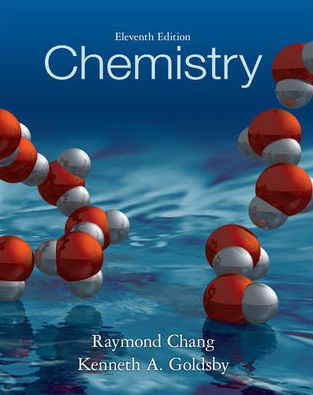### Solution Found!# Determining Ionizable H Atoms in Malonic Acid via Neutralization

Chapter 4, Problem 4.120

(choose chapter or problem)

Get Unlimited Answers! Check out our subscriptions
QUESTION:

The molecular formula of malonic acid is $$\mathrm{C}_{3} \mathrm{H}_{4} \mathrm{O}_{4}$$. If a solution containing 0.762 g of the acid requires 12.44 mL of 1.174 M NaOH for neutralization, how many ionizable H atoms are present in the molecule?

##### Determining Ionizable H Atoms in Malonic Acid via NeutralizationWant To Learn More? To watch the entire video and ALL of the videos in the series:

Explore the process of determining ionizable hydrogen atoms in malonic acid using its molecular formula C3H4O4 and a neutralization reaction with Sodium hydroxide. Through methodical calculations involving the given mass of malonic acid and the required volume of Sodium hydroxide for neutralization we uncover that each molecule of malonic acid contains two ionizable H atoms.

QUESTION:

The molecular formula of malonic acid is $$\mathrm{C}_{3} \mathrm{H}_{4} \mathrm{O}_{4}$$. If a solution containing 0.762 g of the acid requires 12.44 mL of 1.174 M NaOH for neutralization, how many ionizable H atoms are present in the molecule?

Step 1 of 2

The goal is to find the number of ionizable H atoms, present in the molecule.

Given:

$$\begin{array}{l}\text{The molecular formula of malonic acid } =\mathrm{C}_{3} \mathrm{H}_{4} \mathrm{O}_{4}\\ \text{Mass of } \mathrm{C}_{3} \mathrm{H}_{4} \mathrm{O}_{4}=0.762 \mathrm{~g}\\ \text{Volume of } \mathrm{NaOH} \text{ solution } =12.44 \mathrm{~mL}=0.01244 \mathrm{~L}\\ \text{Molarity of } \mathrm{NaOH} \text{ solution } =1.174 \mathrm{M}\end{array}$$

The number of ionizable H atoms in malonic acid can be determined by comparing the moles of NaOH to the moles of malonic acid in 0.762 g.